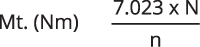# How to select the joints with pins

### NOMOGRAM FOR JOINTS WITH PINS

#### Check the torque:N = power in Hp and n= rpm.
*(N.B. 1 Hp = 0,735 KW)
The nomogram shows in abscissa the values of β x n (deflection angle in degrees for revolutions for minute) and on the ordinate the value of the maximal dynamic load of the joint (Nm), smaller than the statical load in the table. Adding coefficients: Selecting a joint, the maximal value in abscissa is 1200 rpm for joint size till 60.40, down to 250 rpm for the bigger sizes. Multiply the requested torque by one the following adding coefficients.

For a value Angle for Revolutions < 250, the nomogram was calculated for a middle deflection angle of 10°. The value 250 corresponds to 25 rpm only. In these conditions use a security coefficients from 3.5 to 6, depending load and and on a bigger angle.

For a value Angle for Revolutions > 250, use the following coefficients:

LOAD hour/day 3 8 > 8
Uniform 1.75 2.25 2.5
Middle Impulse 2.5 3.5 4
Much Impulse 3.5 5 6Now determin the joint size whase curve is on the point of intersection between the Vertical β x n and the Horizontal through the torque value, expressed in Nm.